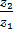# If the complex numbersand the origin form an equilateral triangle, thenis equal to a)b)c)d) |## Question ID - 102849 :- If the complex numbersand the origin form an equilateral triangle, thenis equal to a)b)c)d) |3537

(a)

Letbe the sides of an equilateraland letrepresent the complex numbersrespectivelyFrom the equilateral,andAlso,, since triangle is equilateral. Thus, the complex numbersandhave same modulus and same argument, which implies that the numbers are equal, that isFrom the equilateral

Next Question :